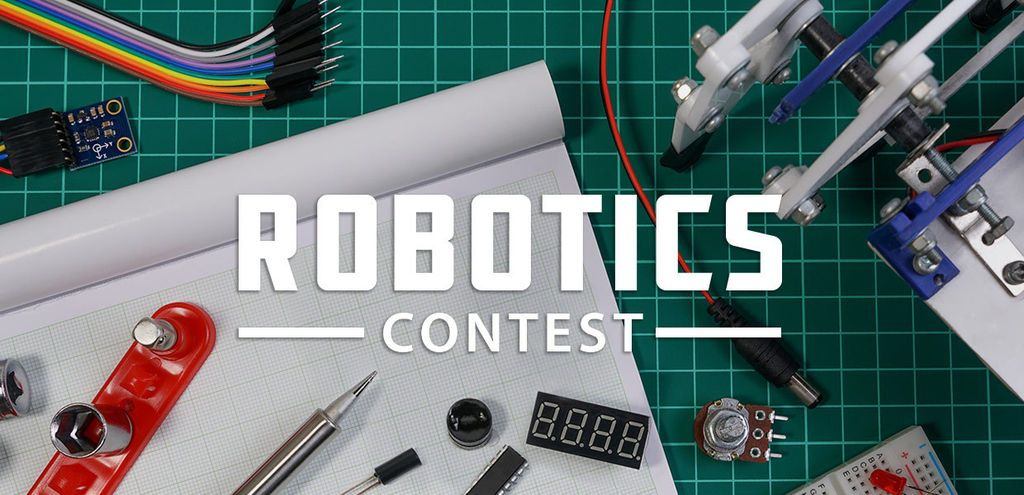Running DC Motor With Arduino Using L298N Motor Driver

399

Hi guys in this instructables we will learn how to drive a motor with arduino. Since arduino can't provide enough powerful signal which could run a dc motor so we need to amplify the signal and convert it to powerful enough to power the dc motor and to do that we have to use a motor driver which will take the signal coming out of arduino and power the motors with adding extra power taken from some battery/ppwers supply.

Teacher Notes

Teachers! Did you use this instructable in your classroom?
Add a Teacher Note to share how you incorporated it into your lesson.

Step 1: Things You Need

For this instructables we will need following things :

Arduino
L298N Motor Driver Module
2 X DC Motors
Joystick Module
12V Battery

Step 2: About Motor Driver

Specification :

The module will allow you to control the speed and direction of two DC motors.
It can control motors which operates between 5 to 35V and up to 2A.
The module has an onboard regulator which helps in giving the output of 5V.
The module can be powered from 5 to 35V from Arduino or external power supply. It is recommended to always use the external voltage supply.
It can also control a stepper motor.

Pin configurations :

Motor A: This terminal block will give the output for the first motor.

12V Jumper: Keep this jumper in place if your supply voltage is less than 12V and the 5V power pin will give you the output of 5V. If the supply voltage is greater than 12V, then remove this jumper and give the 5V supply to the 5V power pin so that the L298 Dual H Bridge IC can work properly.

Power Pins: Give the supply voltage from 5 to 35V at the 12V pin and ground. If your supply voltage is greater than 12, then make sure to remove the 12V jumper. 5V pin will act as Output if the Vs will be less than 12V and 5V pin will act as Input if the Vs will be greater than 12V.

Enable Pins: Remove the jumpers on the Enable A and Enable B if you want to control the speed of DC motors and connect these to PWM pins of Arduino. If you want to control the stepper motor with L298N, then keep the jumper on Enable A and Enable B. Keeping the jumper on these pins means that the these pins will be High.

Logic Pins: Connect the Logic pins to any digital pins of Arduino. These will help in controlling the rotation and speed of DC motors.

Motor B: This terminal block will give the output for the second motor.

5V linear Regulator: This will step down the supply voltage to 5V and will give the output at the 5V pin.

Step 3: Connections

The circuit diagram for connecting the L298N motor driver module with the Arduino is shown in image so do the connections accordingly.

ENA Pin 11
IN1 Pin 9
IN2 Pin 8
IN3 Pin 7
IN4 Pin 6
ENB Pin 10
12V 5 to 12V power supply or battery
GND GND Negative of power supply or battery
In last, connect the two dc motors at the two sides of L298N
For powering the L298N, I have used the 2 rechargeable batteries of 3.7V.

Then connect the Joystick module with the Arduino as follows :

VCC 5V
VER A1
HOR A0
GND GND

Step 4: Code

Please copy the following code and upload it to arduino :

//Joystick Pins
int x_key = A0;
int y_key = A1;
int x_pos;
int y_pos;

//Motor Pins
int EN_A = 11; //Enable pin for first motor
int IN1 = 9; //control pin for first motor
int IN2 = 8; //control pin for first motor
int IN3 = 7; //control pin for second motor
int IN4 = 6; //control pin for second motor
int EN_B = 10; //Enable pin for second motor
//Initializing variables to store data
int motor_speed;
int motor_speed1;

void setup ( ) {
Serial.begin (9600); //Starting the serial communication at 9600 baud rate
//Initializing the motor pins as output
pinMode(EN_A, OUTPUT);
pinMode(IN1, OUTPUT);
pinMode(IN2, OUTPUT);
pinMode(IN3, OUTPUT);
pinMode(IN4, OUTPUT);
pinMode(EN_B, OUTPUT);

//Initializng the joystick pins as input
pinMode (x_key, INPUT) ;
pinMode (y_key, INPUT) ;
}

void loop () {
x_pos = analogRead (x_key) ; //Reading the horizontal movement value
y_pos = analogRead (y_key) ; //Reading the vertical movement value

if (x_pos < 400){ //Rotating the left motor in clockwise direction
motor_speed = map(x_pos, 400, 0, 0, 255); //Mapping the values to 0-255 to move the motor
digitalWrite(IN1, LOW);
digitalWrite(IN2, HIGH);
analogWrite(EN_A, motor_speed);
}

else if (x_pos>400 && x_pos <600){ //Motors will not move when the joystick will be at center
digitalWrite(IN1, LOW);
digitalWrite(IN2, LOW);
}

else if (x_pos > 600){ //Rotating the left motor in anticlockwise direction
motor_speed = map(x_pos, 600, 1023, 0, 255);
digitalWrite(IN1, HIGH);
digitalWrite(IN2, LOW);
analogWrite(EN_A, motor_speed);
}

if (y_pos < 400){ //Rotating the right motor in clockwise direction
motor_speed1 = map(y_pos, 400, 0, 0, 255);
digitalWrite(IN3, LOW);
digitalWrite(IN4, HIGH);
analogWrite(EN_B, motor_speed1);
}

else if (y_pos>400 && y_pos <600){
digitalWrite(IN3, LOW);
digitalWrite(IN4, LOW);
}

else if (y_pos > 600){ //Rotating the right motor in anticlockwise direction
motor_speed1 = map(y_pos, 600, 1023, 0, 255);
digitalWrite(IN3, HIGH);
digitalWrite(IN4, LOW);
analogWrite(EN_B, motor_speed1);
}
}

Step 5: MOTOR RUNNING

So after you upload the code then move the joystick around and as you can see that according to the movement of joystick motors will run accordingly. This can help you make your own rc robot car as well.This is an entry in the
Robotics Contest